## Ambiguous Grammar & Parse Tree-

We have discussed-

• Ambiguous Grammar generates at least one string that has more than one parse tree.
• Parse Tree is the geometrical representation of a derivation.

## Important Points-

### Point-01:

• There always exists a unique parse tree corresponding to each leftmost derivation and rightmost derivation.

 If n parse trees exist for any string w, then there will be-n corresponding leftmost derivations.n corresponding rightmost derivations.

### Point-02:

For ambiguous grammars,

• More than one leftmost derivation and more than one rightmost derivation exist for at least one string.
• Leftmost derivation and rightmost derivation represents different parse trees.

### Point-03:

For unambiguous grammars,

• A unique leftmost derivation and a unique rightmost derivation exist for all the strings.
• Leftmost derivation and rightmost derivation represents the same parse tree.

### Point-04:

• There may exist derivations for a string which are neither leftmost nor rightmost.

### Example

Consider the following grammar-

S → ABC

A → a

B → b

C → c

Consider a string w = abc.

Total 6 derivations exist for string w.

The following 4 derivations are neither leftmost nor rightmost-

#### Derivation-01:

S → ABC

→ aBC    (Using A → a)

→ aBc     (Using C → c)

→ abc     (Using B → b)

#### Derivation-02:

S → ABC

→ AbC    (Using B → b)

→ abC     (Using A → a)

→ abc     (Using C → c)

#### Derivation-03:

S → ABC

→ AbC    (Using B → b)

→ Abc     (Using C → c)

→ abc     (Using A → a)

The other 2 derivations are leftmost derivation and rightmost derivation.

### Point-05:

• Leftmost derivation and rightmost derivation of a string may be exactly same.
• In fact, there may exist a grammar in which leftmost derivation and rightmost derivation is exactly same for all the strings.

### Example

Consider the following grammar-

S → aS / ∈

The language generated by this grammar is-

L = { an , n>=0 } or a*

All the strings generated from this grammar have their leftmost derivation and rightmost derivation exactly same.

Let us consider a string w = aaa.

#### Leftmost Derivation-

S → aS

→ aaS       (Using S → aS)

→ aaaS     (Using S → aS)

→ aaa∈

→ aaa

#### Rightmost Derivation-

S → aS

→ aaS       (Using S → aS)

→ aaaS     (Using S → aS)

→ aaa∈

→ aaa

Clearly,

Leftmost derivation = Rightmost derivation

Similar is the case for all other strings.

### Point-06:

• For a given parse tree, we may have its leftmost derivation exactly same as rightmost derivation.

### Point-07:

• If for all the strings of a grammar, leftmost derivation is exactly same as rightmost derivation, then that grammar may be ambiguous or unambiguous.

### Example

Consider the following grammar-

S → aS / ∈

• This is an example of an unambiguous grammar.
• Here, each string have its leftmost derivation and rightmost derivation exactly same.

Now, consider the following grammar-

S → aS / a / ∈

• This is an example of ambiguous grammar.
• Here also, each string have its leftmost derivation and rightmost derivation exactly same.

Consider a string w = a.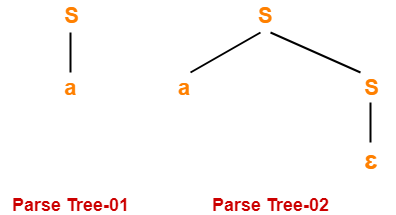Since two different parse trees exist, so grammar is ambiguous.

Leftmost derivation and rightmost derivation for parse tree-01 are-

S → a

Leftmost derivation and rightmost derivation for parse tree-02 are-

S → aS

S → a∈

S → a

Next Article- Language of Grammar

Get more notes and other study material of Theory of Automata and Computation.

Watch video lectures by visiting our YouTube channel LearnVidFun.

## Parse Tree-

• The process of deriving a string is called as derivation.
• The geometrical representation of a derivation is called as a parse tree or derivation tree.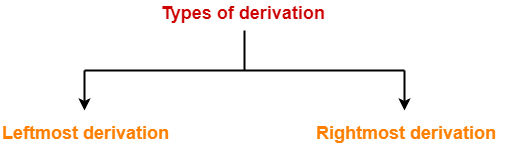## 1. Leftmost Derivation-

• The process of deriving a string by expanding the leftmost non-terminal at each step is called as leftmost derivation.
• The geometrical representation of leftmost derivation is called as a leftmost derivation tree.

### Example-

Consider the following grammar-

S → aB / bA

S → aS / bAA / a

B → bS / aBB / b

(Unambiguous Grammar)

Let us consider a string w = aaabbabbba

Now, let us derive the string w using leftmost derivation.

### Leftmost Derivation-

S   → aB

→  aaBB                   (Using B → aBB)

→ aaaBBB                (Using B → aBB)

→ aaabBB                (Using B → b)

→ aaabbB                (Using B → b)

→ aaabbaBB            (Using B → aBB)

→ aaabbabB            (Using B → b)

→ aaabbabbS          (Using B → bS)

→ aaabbabbbA        (Using S → bA)

→ aaabbabbba         (Using A → a)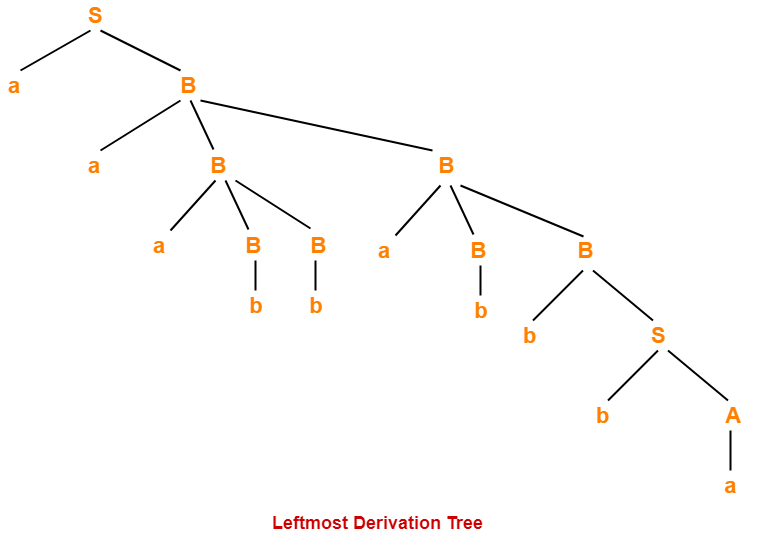## 2. Rightmost Derivation-

• The process of deriving a string by expanding the rightmost non-terminal at each step is called as rightmost derivation.
• The geometrical representation of rightmost derivation is called as a rightmost derivation tree.

### Example-

Consider the following grammar-

S → aB / bA

S → aS / bAA / a

B → bS / aBB / b

(Unambiguous Grammar)

Let us consider a string w = aaabbabbba

Now, let us derive the string w using rightmost derivation.

### Rightmost Derivation-

S   → aB

→  aaBB                    (Using B → aBB)

→ aaBaBB                 (Using B → aBB)

→ aaBaBbS               (Using B → bS)

→ aaBaBbbA             (Using S → bA)

→ aaBaBbba              (Using A → a)

→ aaBabbba              (Using B → b)

→ aaaBBabbba          (Using B → aBB)

→ aaaBbabbba          (Using B → b)

→ aaabbabbba           (Using B → b)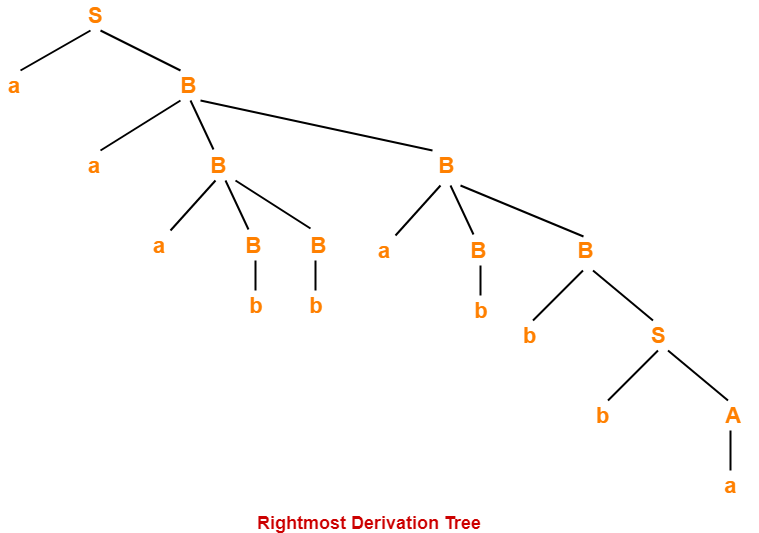### NOTES

• For unambiguous grammars, Leftmost derivation and Rightmost derivation represents the same parse tree.
• For ambiguous grammars, Leftmost derivation and Rightmost derivation represents different parse trees.

Here,

• The given grammar was unambiguous.
• That is why, leftmost derivation and rightmost derivation represents the same parse tree.

 Leftmost Derivation Tree = Rightmost Derivation Tree

## Properties Of Parse Tree-

• Root node of a parse tree is the start symbol of the grammar.
• Each leaf node of a parse tree represents a terminal symbol.
• Each interior node of a parse tree represents a non-terminal symbol.
• Parse tree is independent of the order in which the productions are used during derivations.

## Yield Of Parse Tree-

• Concatenating the leaves of a parse tree from the left produces a string of terminals.
• This string of terminals is called as yield of a parse tree.

## Problem-01:

Consider the grammar-

S → bB / aA

A → b / bS / aAA

B → a / aS / bBB

For the string w = bbaababa, find-

1. Leftmost derivation
2. Rightmost derivation
3. Parse Tree

## Solution-

### 1. Leftmost Derivation-

S   → bB

→ bbBB              (Using B → bBB)

→ bbaB              (Using B → a)

→ bbaaS            (Using B → aS)

→ bbaabB          (Using S → bB)

→ bbaabaS        (Using B → aS)

→ bbaababB      (Using S → bB)

→ bbaababa       (Using B → a)

### 2. Rightmost Derivation-

S   → bB

→ bbBB                (Using B → bBB)

→ bbBaS              (Using B → aS)

→ bbBabB            (Using S → bB)

→ bbBabaS          (Using B → aS)

→ bbBababB        (Using S → bB)

→ bbBababa        (Using B → a)

→ bbaababa         (Using B → a)

### 3. Parse Tree-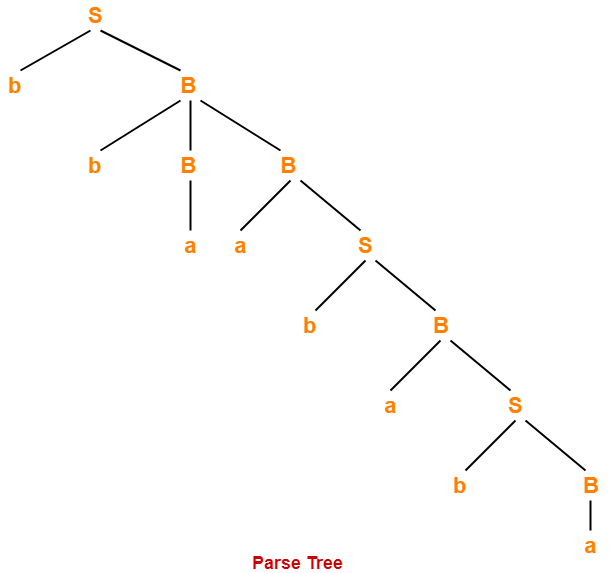• Whether we consider the leftmost derivation or rightmost derivation, we get the above parse tree.
• The reason is given grammar is unambiguous.

## Problem-02:

Consider the grammar-

S → A1B

A → 0A / ∈

B → 0B / 1B / ∈

For the string w = 00101, find-

1. Leftmost derivation
2. Rightmost derivation
3. Parse Tree

## Solution-

### 1. Leftmost Derivation-

S   → A1B

→ 0A1B              (Using A → 0A)

→ 00A1B            (Using A → 0A)

→ 001B              (Using A → ∈)

→ 0010B            (Using B → 0B)

→ 00101B          (Using B → 1B)

→ 00101             (Using B → ∈)

### 2. Rightmost Derivation-

S   → A1B

→ A10B                (Using B → 0B)

→ A101B              (Using B → 1B)

A101                (Using B → ∈)

→ 0A101              (Using A → 0A)

→ 00A101            (Using A → 0A)

→ 00101               (Using A → ∈)

### 3. Parse Tree-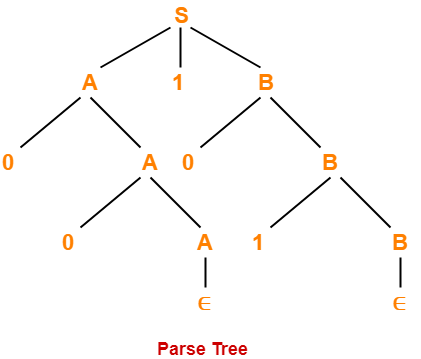• Whether we consider the leftmost derivation or rightmost derivation, we get the above parse tree.
• The reason is given grammar is unambiguous.

To gain better understanding about Derivations and Parse Tree,

Watch this Video Lecture

Next Article- Elimination of Left Recursion

Get more notes and other study material of Theory of Automata and Computation.

Watch video lectures by visiting our YouTube channel LearnVidFun.